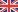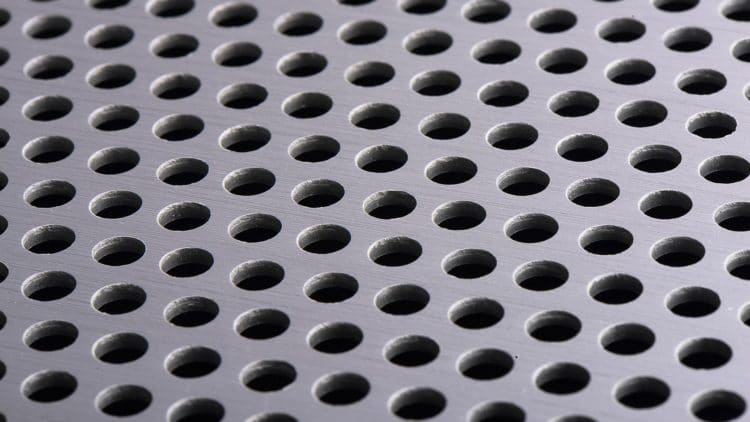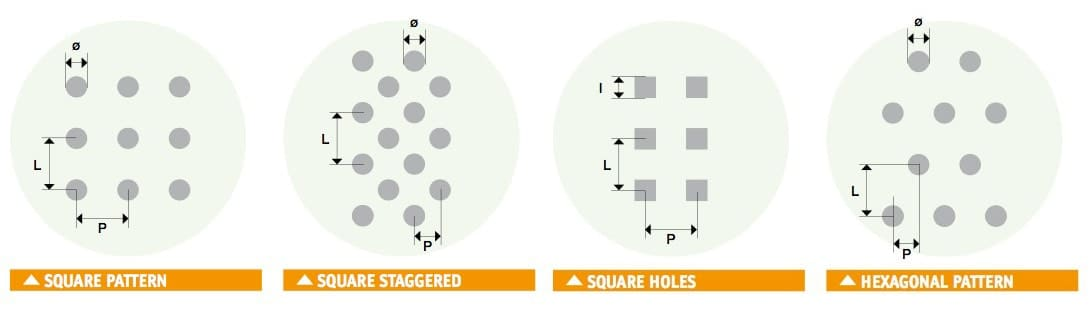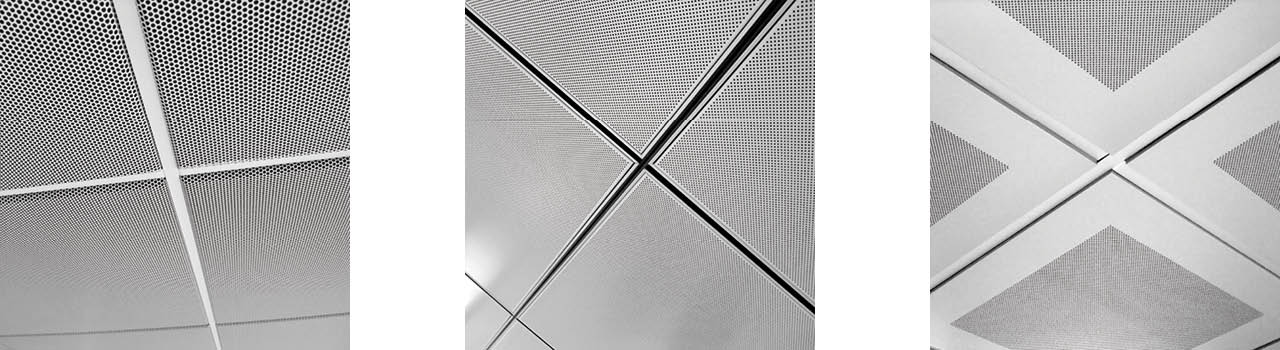Change language

# How to calculate the productivity of a perforation line for metal ceilings

May 2017 UncategorizedPerforated sheet metal is gaining popularity in several industries: for lighting and sun-shading applications, facades, perforated roller doors but especially for soundproofing applications, namely sheet metal walls and ceilings.

Ceiling tiles measuring 2’x2′ or 600x600mm are produced today in gypsum and mineral fiber, but perforated sheet metal tiles are gaining popularity in all markets.

The perforation of a sheet metal, however, would be a time consuming operation with standard CNC punch presses, and the quantities are considerable. For this reasons, metal ceilings producers prefer to adopt dedicated lines, that use hard tools performing up to 1000 holes per each hit, in order to reach a sufficient productivity.

The following video shows a complete system for the metal tiles perforation and bending.

In this article, I will show how to calculate the productivity of a perforating line, given the perforation pattern, the force available in the press and the hits per minute available.

Follow the link and discover more on perforating lines for metal coils.

## Perforation pattern

The following picture shows possible common perforating patterns:The most common patterns are the square and square staggered patterns, and by the way they both can be obtained with the same perforating tool, just removing half of the punches.

Hole diameters can be as small as 0,7mm up to 3mm and more. The most common dimensions go from 1,5mm to 2,5mm and the distance between the holes goes generally from 4 to 6mm.

In the following calculations, dimensions will be expressed in [mm] and forces in [N].

## Calculating the maximum pitch of the perforating die

The dimension of the holes is necessary to calculate the required cutting force. To complete the calculation, we need to know the sheet metal thickness and material type.

Ceiling tiles are generally made out of:

mild steel (cutting force parameter CFP = 400N/mm2) or
aluminum (CFP = 250N/mm2)

and the thickness goes from 0,4 to 0,8mm.

The force required to cut each hole is calculated as follows:

• Force per hole = (Hole perimeter) * (Metal thickness) * CFP

And for circular holes:

• Force per hole = 3,14 * (Hole diameter) * (Metal thickness) * CFP

For example, a hole diameter 2mm on an aluminum tile with thickness 0,6mm requires 942 N for cutting.

Now, we need to find how many holes are in each perforated row, knowing the perforated width and distance between the holes.

• Holes per row = (Perforated width) / (Distance between the holes)

For example, leaving in the metal tile a frame of 20mm of non-perforated area, the perforated width results 560mm and with 5mm distance between the holes we find a total of 112 holes per row.

Now we can calculate the total force per row.

• Total force per row = (Holes per row) * (Force per hole)

In our example, the cutting force per row is 105500N (about 10 tons).

Knowing the total force available in the press, we can calculate the maximum number of rows that can be punched on the press.

• Total number of rows = (Press force) / (Total force per row)

If we consider the total available force of 600kN, in our example the maximum number of rows is:

(Total number of rows) = 600000N / 105500N = 5,68 => 5 rows

We have to take only the entire part of the result. Even if we seem to be close to the press maximum force, consider that quite often the height of the punches is staggered, reducing the required cutting force by half.

In our example, I will consider using just four rows. In a square pattern, with hole distance of 5mm (see picture), four rows lead to calculating a pitch of:

• Die pitch = (Number of rows) * (distance between the rows)

(Die pitch) = 5mm * 4 = 20mm

## Calculating the production speed of the perforating line

With the die pitch and the speed of the press (in hits per minute), we can calculate the time for perforating a metal tile.

• Perforating time in seconds = 60 * (Perforated length) / (Die Pitch) / (Hits per minute)

And in our example:

(Perforating time in seconds) = 60 * (560mm) / (20mm) / (150 hits per minute) = 11,2 seconds

To calculate now the production time for a tile, we need to add the corner notching time and the time for shearing. Let’s consider 2 seconds per each one of these operations.

• Production time = (Perforating time) + (Notching time) + (cutting time)

(Production time per tile) = 11,2s + 2s+ 2s = 15,2 seconds

The line in our example will produce approximately four panels per minute. Of course, any reduction of the pitch will modify the production time.Note: 3 panels 600x600mm correspond to 1,08 square meter, and this helps to quickly calculate the capacity of the line in square meters per shift. In one line like the one in the example, producing 4 panels per minute, we can consider the line efficiency 75% (time for coil change and line setup, etc) and a total production rate of 1 square meter of metal ceiling per minute.

## Conclusions

In the management of a perforating line, knowing the parameters and capacity of the line ensures the reliability of the machine, smooth production, longer durability of the dies and reduced maintenance cost.

I believe that knowing how to calculate the productivity of a production line helps the production manager to take quick decision on the shop floor. The best way to calculate the productivity is to prepare a table with the maximum pitch available for every die set and material thickness on a given machine, and use this along with the last two formulas for calculating the productivity and running time of the line.

Author
Andrea Dallan – C.E.O.